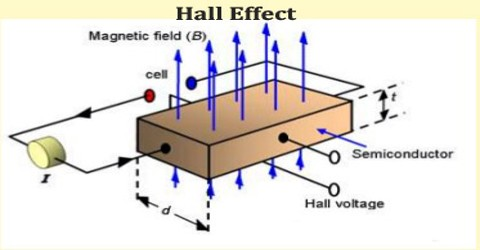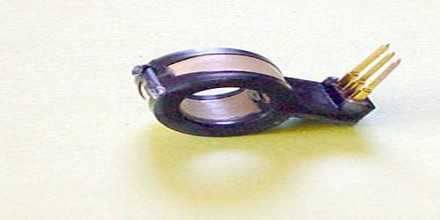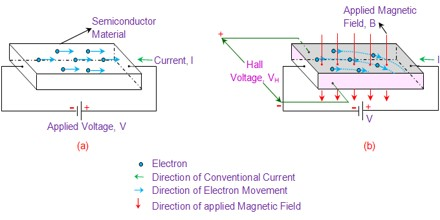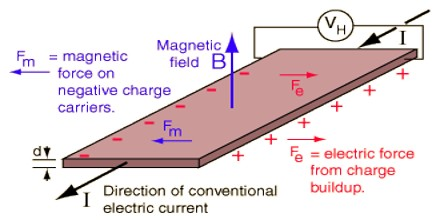Physics

# Hall EffectHall Effect

Definition

Hall Effect is a direct result of Lorentz forces acting on the charges in the current, and is named after U.S. physicist Edwin Herbert Hall (1855-1938). It is development of a transverse electric field in a solid material when it carries an electric current and is placed in a magnetic field that is perpendicular to the current.

The Hall coefficient is defined as the ratio of the induced electric field to the product of the current density and the applied magnetic field. It is a characteristic of the material from which the conductor is made, since its value depends on the type, number, and properties of the charge carriers that constitute the current.The electric field, or Hall field, is a result of the force that the magnetic field exerts on the moving positive or negative particles that constitute the electric current. Whether the current is a movement of positive particles, negative particles in the opposite direction, or a mixture of the two, a perpendicular magnetic field displaces the moving electric charges in the same direction sideways at right angles to both the magnetic field and the direction of current flow. The accumulation of charge on one side of the conductor leaves the other side oppositely charged and produces a difference of potential. An appropriate meter may detect this difference as a positive or negative voltage. The sign of this Hall voltage determines whether positive or negative charges are carrying the current.

Applications of Hall Effect

Hall Effect describes the behavior of free charge carriers in the semiconductor when subjected to the electric and magnetic fields. It is well known that a potential applied across the semiconductor material causes the electrons within it to move in the direction opposite to that of the applied field. Further, it is to be noted that, in this case, the path of the electrons will be almost a straight line.The direction of the Hall voltage so developed will be perpendicular to both the direction of current flow as well as to the applied magnetic field. Thus, the Hall Effect can be stated as the phenomenon where the current carrying conductor or semiconductor subjected to the external magnetic field develops a voltage across its terminals in the direction perpendicular to both the flow of current as well as to the direction of applied magnetic field.

Uses of Hall Effect

Hall Effect is a very useful phenomenon and helps to –

Determine the Type of Semiconductor:

By knowing the direction of the Hall Voltage, one can determine that the given sample is whether n-type semiconductor or p-type semiconductor. This is because Hall coefficient is negative for n-type semiconductor while the same is positive in the case of p-type semiconductor.

Determine the Mobility (Hall Mobility):

Mobility expression for the electrons (μn) and the holes (μp), expressed in terms of Hall coefficient is given by,

μn = σnRH and μp = σpRH

Where, σn and σp represent the conductivity due to the electrons and the holes, respectively.Quantum Hall Effect:

For a two-dimensional electron system which can be produced in a MOSFET, in the presence of large magnetic field strength and low temperature, one can observe the quantum Hall effect, in which the Hall conductance σ undergoes quantum Hall transitions to take on the quantized values.

Spin Hall Effect:

The spin Hall Effect consists in the spin accumulation on the lateral boundaries of a current-carrying sample. No magnetic field is needed. It was predicted by M. I. Dyakonov and V. I. Perel in 1971 and observed experimentally more than 30 years later, both in semiconductors and in metals, at cryogenic as well as at room temperatures.

Anomalous Hall Effect:

In ferromagnetic materials and paramagnetic materials in a magnetic field, the Hall resistivity includes an additional contribution, known as the anomalous Hall effect, which depends directly on the magnetization of the material, and is often much larger than the ordinary Hall effect.